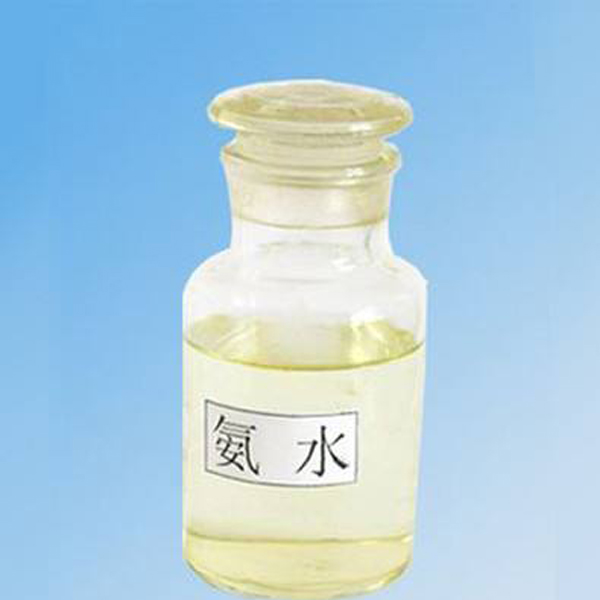# 氨水的特性

2019-12-23 15:54:21

1.氨水的挥发性

2.氨水的腐蚀性

3.氨水的弱碱性

NH3+H2O===NH3·H2O（可逆反应）

NH3·H2O===NH4+ +OHˉ（可逆反应）①能使无色酚酞试液变红色，能使紫色石蕊试液变蓝色，能使湿润红色石蕊试纸变蓝。实验室中常用此法检验NH3的存在。

②能与酸反应，生成铵盐。浓氨水与挥发性酸(如浓盐酸和浓硝酸)相遇会产生白烟。

NH3+HCl===NH4Cl (白烟)

NH3+HNO3===NH4NO3 (白烟)

SO2+2NH3·H2O===(NH4)2SO3+H2O

(NH4)2SO3+SO2+H2O===2NH4HSO3

4.氨水的不稳定性

NH3·H2O===NH3↑+H2O

5.氨水的沉淀性

Al3﹢ +3NH3·H2O===Al(OH)3↓+3NH4+

Fe2﹢ +2NH3·H2O===Fe(OH)2↓+2NH4+

4Fe(OH)2+O2+2H2O===4Fe(OH)3（红褐色）

6.氨水的络合性

Ag2O+4NH3·H2O===2[Ag(NH3)2]﹢ +2OHˉ+3H2O

Zn(OH)2+4NH3·H2O===[Zn(NH3)4]2﹢+2OHˉ+4H2O

Cu(OH)2+4NH3·H2O===[Cu(NH3)4]2+(深蓝色) +2OHˉ+4H2O

7.氨水的还原性

3Cl2+8NH3·H2O===6NH4Cl+N2+8H2O